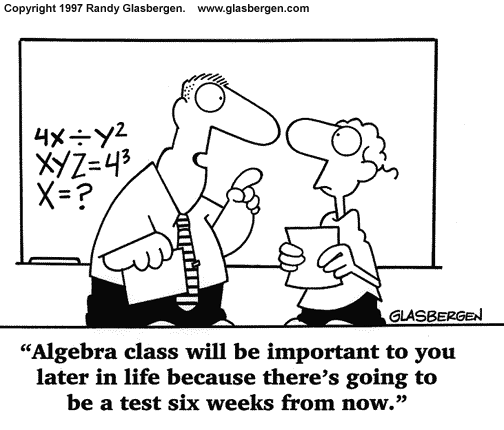## N5 Unit 3 Outcome 1.1 – PracticeN5 Unit 3 Practice – Outcome 1

Click on image to enlarge.

😉

## N5 Unit 2 Outcome 1.5 – PracticeClick on image to enlarge.

😉

## N5 Unit 2 Outcome 1.1 – PracticeClick on image to enlarge.

😉

## Algebraic OperationsIn this topic you will learn how to:

• multiply algebraic expressions involving brackets
• factorise algebraic expressions
• factorise trinomial expressions

Class exercises: Int2 Unit 1 – Algebraic Operations

Homework: Outcome 1.4 – Algebraic Operations – Due date TBA (will be issued in August)

If you have missed any of the topic or would like extra explanation and practice please look at these revision notes from the Maths4Scotland Int2 website:

Algebra Practice

Brackets & Equations

Difference of Two Squares

Factorising Trinomials

Please comment below if you have any questions about the homework exercise or the topic.

.

## Algebraic Functions and GraphsNew Topic: Algebraic Functions and Graphs

By the end of this topic you will be able to:

• complete the square for quadratic functions

• recognise the probable form of a function from its graph

• draw graphs of related functions given f(x), f(x) being a simple polynomial or trigonometric function

• know the general features of the graphs of y=ax and y=logax

## FactorsNew Topic: Factors, MiA 3³ Ch5

By the end of this topic you will be able to:

• by first looking for a common factor
• by recognising a difference of two squares
• trinomials (e.g. x² -2x -3 and 2x² – 7x + 3)
• ## Equations & InequationsNew Topic: Equations & Inequations, MiA 4³ Ch5

By the end of this topic you will have revised your basic algebra skills including:

• gathering like terms & simplifying expressions
• substituting values (including negative numbers)
• evaluating expressions
• multiplying out brackets (single and double)
• solving equations
• You will be able to solve:

• equations involving expanding double brackets
• equations with fractions
• inequations ( etc)
• ## Brackets & EquationsNew Topic: Brackets & Equations, MiA 3³ Ch3

By the end of this topic you will have revised your basic algebra skills including:

• gathering like terms & simplifying expressions
• substituting values (including negative numbers)
• evaluating expressions
• multiplying out brackets
• You will be able to:

• multiply out (expand) double brackets
• expand squared brackets
• ## Simultaneous EquationsNew Topic: Simultaneous Equations, MiA 3³ Ch13

By the end of this topic you will be able to:
• solve simultaneous equations in two unknowns
(i) graphically
(ii) by substitution
(iii) by elimination
• solve problems using simultaneous equations

## Equations and InequationsNew Topic: Equations & Inequations, MiA 4² Ch6

By the end of this topic you will be able to solve equations involving variables on both sides, equations with brackets and inequalities.